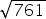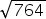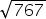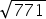Mathematics
Easy

Question

# In a right triangle, one side is 19 m, the second side is 20 m. Find the hypotenuse.

##Hint:

## The correct answer is:### Here, we have to find the hypotenuse.In a right triangle, the two other sides are 19m and 20m.We have, h² = 19² + 20²Or, h² = 361+400Or, h = √761 m.Hence, the correct option is (A) .

TThe pythagoras theorem is expressed as h2 = p+ b2 ,where h is the hypotenuse and p &b are the other two sides.

### Related Questions to study#### With Turito Foundation.#### Get an Expert Advice From Turito.